StatLect

# Choice of a regularization parameter

This lecture discusses how to choose the regularization parameter of a linear regression using train-validation-test splits.## Preliminaries

In our first attempts at building predictive models (with the inflation data set), we estimated linear regression models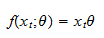where the inputis arow vector and the parameteris a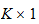vector of regression coefficients.

We used (through the implementation of linear regression in scikit-learn) the ordinary least squares (OLS) estimatorwhere the matrixand the vectorare obtained by stacking inputs and outputs vertically.

The OLS estimator is the analytical solution of the empirical risk minimization problem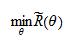where the empirical riskis the mean squared error (MSE) on the training sample.

Under certain assumptions, OLS is the estimator having the lowest MSE among those that have zero bias (see the lecture on the Gauss-Markov theorem).

But why restricting ourselves to zero bias estimators?

Our objective is to minimize MSE, so we can accept some bias if the final result is to reduce MSE.

## Ridge estimator

We now introduce the Ridge estimator, a biased estimator that can have lower MSE than the OLS estimator:whereis a positive scalar called a regularization parameter (a positive scalar) andis the identity matrix.

The Ridge estimator is the analytical solution of the regularized empirical risk minimization problem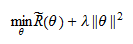where the empirical riskis the MSE on the training sample and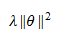is a penalty for model complexity (large positive or negative values of the parameters), called a regularization term.

## There always exists a Ridge estimator that is better than the OLS estimator

It has been proved by Theobald (1974) and Farebrother (1976) that there always exists a value of the regularization parametersuch that the Ridge estimator has lower risk (as measured by the population MSE) than the OLS estimator.

Note that we are talking about the true risk, not the empirical risk on the training sample, which is a biased estimate of true risk.

## How to choose the regularization parameter

How do we choose the regularization parameter?

Now that we know how to work with train-val-test splits, we can choose the regularization parameteras follows:

• on the training set, we estimate several different Ridge regressions, with different values of the regularization parameter;

• on the validation set, we choose the best model (the regularization parameter which gives the lowest MSE on the validation set);

• on the test set, we check how much overfitting we have done by doing model selection on the validation set.

## Python example: inflation data set

In our Python example, we continue to use the same inflation data set used previously.

### Import the data and use scikit-learn to split into train-val-test (60-20-20)

We first import the data and split it into train-val-test.

# Import the packages used to load and manipulate the data
import numpy as np # Numpy is a Matlab-like package for array manipulation and linear algebra
import pandas as pd # Pandas is a data-analysis and table-manipulation tool

# Import the function that performs sample splits from scikit-learn
from sklearn.model_selection import train_test_split

try:
except:
y = y.values # Transform y into a numpy array

# Print some information about the output variable
print('Class and dimension of output variable:')
print(type(y))
print(y.shape)

# Load the input variables with pandas
try:
except:
x = x.values

# Print some information about the input variables
print('Class and dimension of input variables:')
print(type(x))
print(x.shape)

# Create the training sample
x_train, x_val_test, y_train, y_val_test
= train_test_split(x, y, test_size=0.4, random_state=1)

# Split the remaining observations into validation and test
x_val, x_test, y_val, y_test
= train_test_split(x_val_test, y_val_test, test_size=0.5, random_state=1)

# Print the numerosities of the three samples
print('Numerosities of training, validation and test samples:')
print(x_train.shape, x_val.shape, x_test.shape)

The output is:

Class and dimension of output variable:
class 'numpy.ndarray'
(270, 1)
Class and dimension of input variables:
class 'numpy.ndarray'
(270, 113)
Numerosities of training, validation and test samples:
162 54 54

### Estimate and validate the OLS regression with all inputs

We re-estimate the OLS regression with all the 113 input variables, so we can use its performance as a benchmark.

# Import functions from scikit-learn
from sklearn import linear_model # Linear regression
from sklearn.metrics import mean_squared_error, r2_score # MSE and R squared

# Create linear regression object
lr = linear_model.LinearRegression()

# Train the model using the training set
lr.fit(x_train, y_train)

# Make predictions on the training and validation sets
y_train_pred = lr.predict(x_train)
y_val_pred = lr.predict(x_val)

# Print empirical risk on both sets
print('MSE on training set:')
print(mean_squared_error(y_train, y_train_pred))
print('MSE on validation set:')
print(mean_squared_error(y_val, y_val_pred))
print('')

# Print R squared on both sets
print('R squared on training set:')
print(r2_score(y_train, y_train_pred))
print('R squared on validation set:')
print(r2_score(y_val, y_val_pred))

The output is:

MSE on training set:
0.014398812247239373
MSE on validation set:
0.16729075969537868

R squared on training set:
0.9084301866005341
R squared on validation set:
0.2922871496151206

### Search for the best ridge model

In the following code:

• we estimate several Ridge regression models (with different values of the regularization parameter) on the training set;

• we perform model selection, choosing the Ridge regression that has the best performance on the validation set;

• we check the performance of the chosen model on the test set.

# Save MSE on validation set of unregularized regression
MSE = mean_squared_error(y_val, y_val_pred)

# Set up grid for regularization parameter
exponents = np.arange(1,300)
lambdas = 10 * 0.90 ** exponents

# Estimate Ridge regression for each regularization parameter in grid
# and save if performance on validation is better than that of
# previous regressions
for lambda_j in lambdas:
lr_j = linear_model.Ridge(lambda_j, normalize=True)
lr_j.fit(x_train, y_train)
y_val_pred_j = lr_j.predict(x_val)
MSE_j = mean_squared_error(y_val, y_val_pred_j)
if MSE_j < MSE:
lr = lr_j
MSE = MSE_j

# Make predictions on the train, validation and test sets
y_train_pred = lr.predict(x_train)
y_val_pred = lr.predict(x_val)
y_test_pred = lr.predict(x_test)

# Print empirical risk on all sets
print('MSE on training set:')
print(mean_squared_error(y_train, y_train_pred))
print('MSE on validation set:')
print(mean_squared_error(y_val, y_val_pred))
print('MSE on test set:')
print(mean_squared_error(y_test, y_test_pred))
print('')

# Print R squared on all sets
print('R squared on training set:')
print(r2_score(y_train, y_train_pred))
print('R squared on validation set:')
print(r2_score(y_val, y_val_pred))
print('R squared on test set:')
print(r2_score(y_test, y_test_pred))

The output is:

MSE on training set:
0.021890591243460565
MSE on validation set:
0.10386114114319608
MSE on test set:
0.12027367573648916

R squared on training set:
0.860785923106124
R squared on validation set:
0.5606220906849759
R squared on test set:
0.4199777763134154

With Ridge regressions, we managed to significantly reduce overfitting on the training set, although overfitting is still severe.

There is some overfitting also on the validation set, but we did much better than with the previously attempted model selection method (random selection of subsets of regressors). Why? Here we used the validation set to select a single parameter (). Instead, when we chose the best model from a large set of randomly generated ones, we were basically using the validation sample to set more than a hundred parameters (all the regression coefficients), which induced a lot of overfitting also on the validation set.

### References

Farebrother, R. W. (1976) " Further results on the mean square error of ridge regression", Journal of the Royal Statistical Society, Series B (Methodological), 38, 248-250.

Theobald, C. M. (1974) " Generalizations of mean square error applied to ridge regression", Journal of the Royal Statistical Society, Series B (Methodological), 36, 103-106.